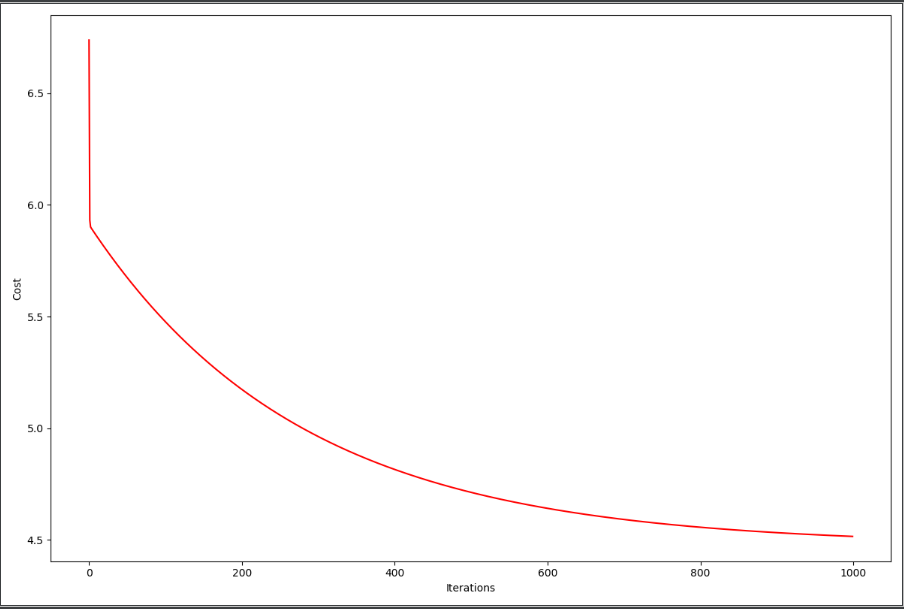# 【Python】机器学习之单变量线性回归 利用批量梯度下降找到合适的参数值

【Python】机器学习之单变量线性回归 利用批量梯度下降找到合适的参数值

1、导包

import numpy as np
import pandas as pd
import matplotlib.pyplot as plt

2、之前写的CostFunction函数

def computeCost(X, y, theta):
inner = np.power(((X * theta.T) - y), 2)
return np.sum(inner) / (2 * len(X))

3、引入文件，把X和Y分开，在X左边加一列1，θ0和θ1设置为0，0

path = 'ex1data1.txt'
data = pd.read_csv(path, header=None, names=['Population', 'Profit'])
data.insert(0, 'Ones', 1)rows = data.shapecols = data.shapeX = data.iloc[:, 0:cols - 1]Y = data.iloc[:, cols - 1:cols]theta = np.mat('0,0')X = np.mat(X.values)Y = np.mat(Y.values)cost = computeCost(X, Y, theta)

4、设置更新速率α为0.01，设置迭代次数为1000次

alpha = 0.01
iters = 1500

5、写出梯度下降函数的实现

def gradientDescent(X, Y, theta, alpha, iters):
temp = np.mat(np.zeros(theta.shape))  # 一个数组，temp大小为θ的个数
parameters = int(theta.ravel().shape)  # 参数的个数
cost = np.zeros(iters)  # 一个数组，存着每次计算出来的costFunction的值

for i in range(iters):
error = (X*theta.T)-Y;  #误差值
for j in range(parameters):
term = np.multiply(error,X[:, j])
temp[0,j] = theta[0,j] - ((alpha/len(X)) * np.sum(term))
theta = temp
cost[i] = computeCost(X,Y,theta)
return theta, cost

temp数组存的是临时变量，因为所有的θ需要同步更新，所以先存入临时变量中，后面计算完所有θ的值后再同步更新。

parameters是一个int值的数，即有多少个变量，本题中有θ0和θ1，所以parameters=2

cost是一个数组，大小和迭代次数一样，每一层存放当前迭代次数下的CostFunction的返回值

6、调用函数，并返回结果

g, cost = gradientDescent(X, Y, theta, alpha, iters)
print(g)

7、把图打出来，看看是否收敛

fig, ax = plt.subplots(figsize=(12,8))
ax.plot(np.arange(iters),cost,'r')
ax.set_xlabel('Iterations')
ax.set_ylabel('Cost')
plt.show()PS：数据集在机器学习的第一篇中的最下方。

posted @ 2019-10-04 17:37  秦羽纶  阅读(439)  评论(0编辑  收藏